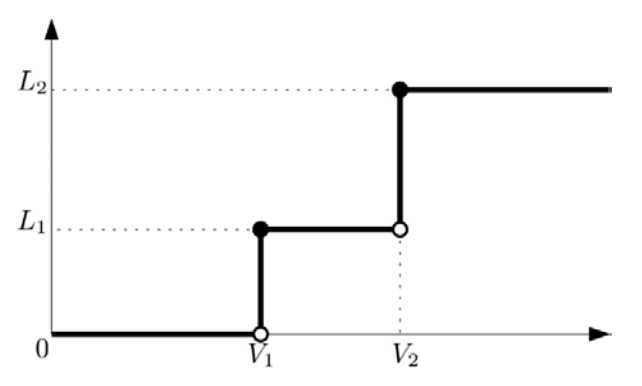시간 제한메모리 제한제출정답맞힌 사람정답 비율
1.3 초 512 MB7161149916.981%

## 문제

A Light-Emitting Diode (LED) is a semiconductor light source, which emits light when an electric current of voltage higher than a threshhold is applied to its leads. ACM R&D recently reported that they have succesfully developed a new LED, namely, ACMOLED. An ACMOLED has a special behavior that the intensity of light emitted from it changes in two steps as the voltage of the electric current increases, as depicted in the graph below.As shown, an ACMOLED is not activated in the voltage range from 0 to V1, while it emits light with intensity L1 ≥ 0 when the voltage reaches the first threshold V1 and light with intensity L2L1 when the voltage reaches the x threshold V2. More specifically, if F(v) is the function that maps voltage v to the intensity of light emitted from an ACMOLED, then for four real numbers L1, L2, V1, and V2 with 0 ≤ L1L2 and 0 < V1 < V2, we have

F(v) = 0 (if 0 ≤ v < V1), L1 (if V1v < V2), L2 (if vV2)

The very issue now is that ACM R&D still does not know the exact values of two threshold voltage values V1 and V2 and the two intensity values L1 and L2 as well. Researchers in ACM R&D plan to estimate these four values for ACMOLEDs by repeated experiments.

Experiments are performed by applying current of a specific voltage and observing the intensity of light emitted from an ACMOLED. After n repeated experiments with different voltage values, obtained are the data of n tuples (v1, l1), (v2, l2), ..., (vn, ln), where li is the observed intensity for voltage vi. Due to the impreciseness of the observing device and other reasons, the experimental data are not accurate and may contain some error. Nonetheless, they want to find a best estimated intensity function F(v) that minimizes the following error function:

error(F) = max1≤in |li - F(vi)|

where |x| denotes the absolute value of a real number x.

For a given data of n tuples, write a program that finds an estimated intensity function F that minimizes the above error function and outputs the value of error(F).

## 입력

Your program is to read from standard input. The input starts with a line containing an integer n (1 ≤ n ≤ 300,000), where n is the number of tuples (vi, li) in the experimental data. In the following n lines, each line contains two integers, which range inclusively from 0 to 109, representing vi and li in each tuple (vi, li) of the experimental data. Note that you may assume that there are no two tuples (vi, li) and (vj, lj) in the input such that 1 ≤ i < jn and vi = vj.

## 출력

Your program is to write to standard output. Print exactly one line consisting of one real number, rounded to the first decimal place, which represents the minimum value of error(F).

## 예제 입력 1

5
0 0
2 1
3 5
6 7
7 11


## 예제 출력 1

1.0


## 예제 입력 2

10
5 9
8 9
0 0
23 18
26 18
2 0
3 0
13 9
18 9
21 18


## 예제 출력 2

0.0